### 游戏介绍

#### 规则介绍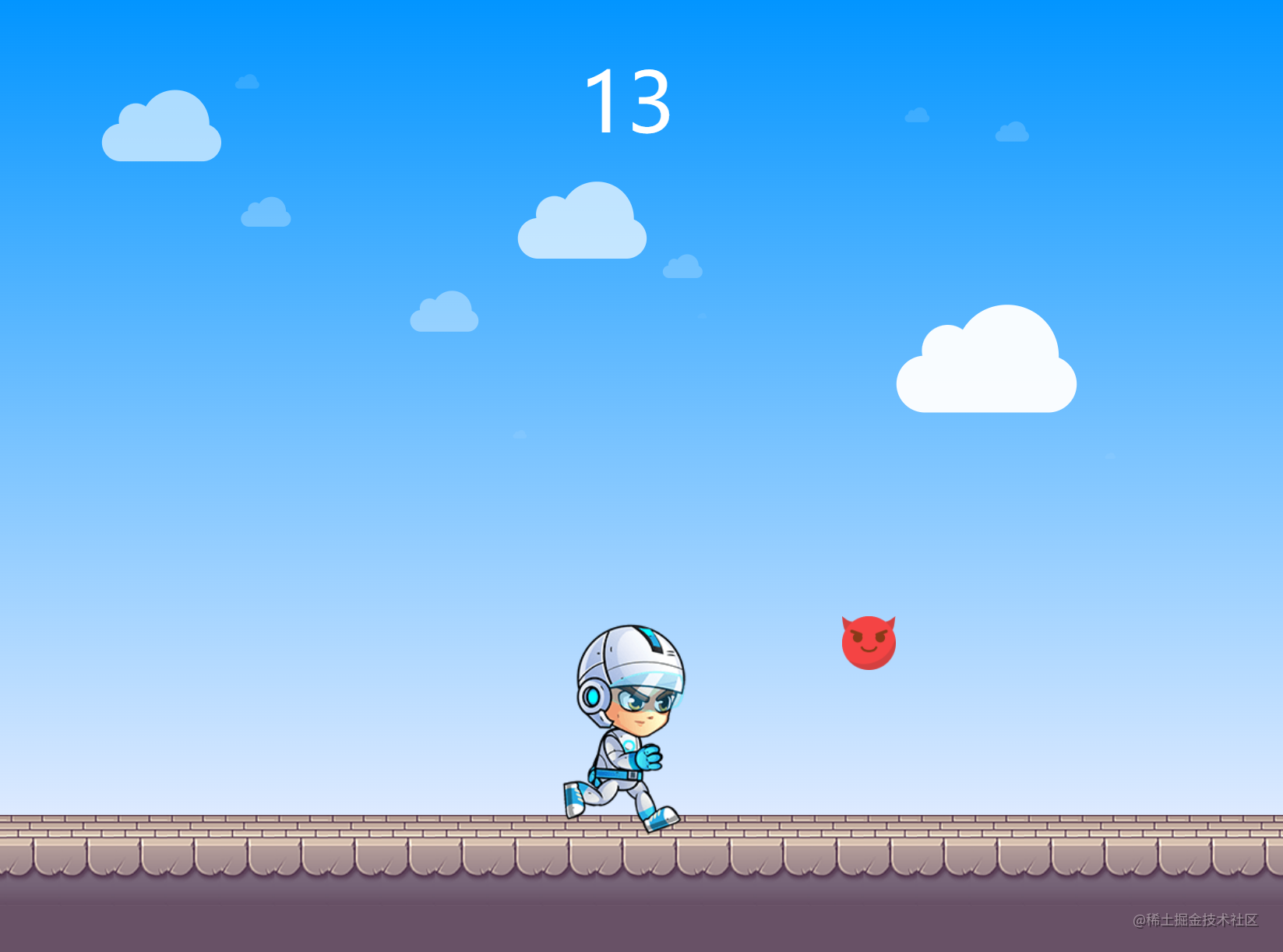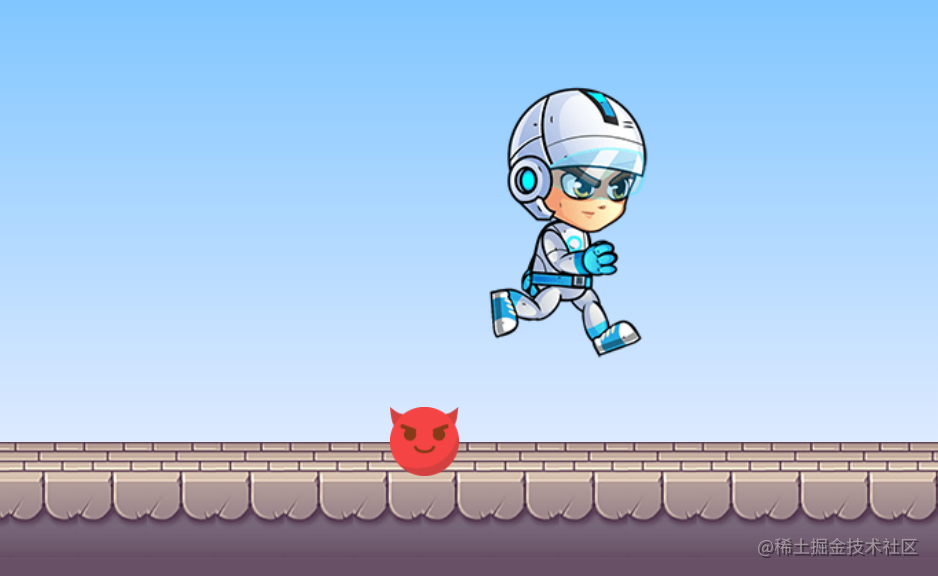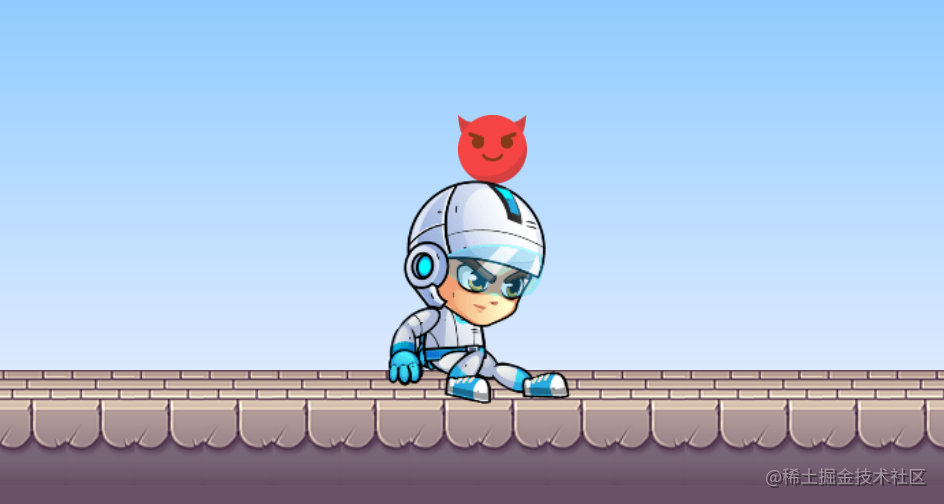### 游戏开发

#### 场景开发

##### 白云开发``````.cloud-item {
position: absolute;
width: 175px;
height: 55px;
margin: 50px;
background: #fff;
}
.cloud-item::before, .cloud-item::after {
content: '';
display: block;
background: #fff;
position: absolute;
}
.cloud-item::before {
content: '';
display: block;
width: 100px;
height: 100px;
top: -90%;
right: 10%;
}
.cloud-item::after {
content: '';
display: block;
width: 50px;
height: 50px;
top: -54%;
left: 14%;
transform: rotate(-25deg);
}
````````````<div class="cloud-wrap" ref="cloudWrap"></div>
``````
``````screenWidth: document.documentElement.clientWidth,
lastCreateTime: 0,
cloudFrequency: 10,
cloudSpeed: 1

// 生成云朵
createCloud() {
let now = new Date().getTime()
if (now - this.lastCreateTime > 3000) {
// 创建云朵
let cloudItem = document.createElement('div')
cloudItem.className = 'cloud-item'
// 设置云朵变化系数
cloudItem.cloudScale = Math.random()
// 设置云朵大小
cloudItem.style.transform = 'scale('+cloudItem.cloudScale+')'
// 设置云朵透明度
cloudItem.style.opacity = cloudItem.cloudScale
// 设置云朵位置
let _left = this.screenWidth
cloudItem.style.left = _left + 'px'
let _top = Math.random() * 400
cloudItem.style.top = _top + 'px'

this.\$refs.cloudWrap.appendChild(cloudItem)
// 云朵移动
let cloudMove = () => {
// 云朵越大，移动速度越快
let moveX = this.cloudSpeed * cloudItem.cloudScale
let _left = +cloudItem.style.left.slice(0, -2)
cloudItem.style.left = _left - moveX + 'px'

// 如果云朵距离屏幕顶部距离大于等于屏幕高度，则移除此云朵
if (cloudItem.offsetLeft < (-cloudItem.offsetWidth)) {
this.\$refs.cloudWrap.removeChild(cloudItem)
} else {
requestAnimationFrame(cloudMove)
}
}
cloudMove()
cloudMove()
this.lastCreateTime = now
}
requestAnimationFrame(this.createCloud)
},
``````

https://code.juejin.cn/pen/7103402725735399438

##### 地面开发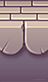``````<!-- 地面 -->
<div class="ground-wrap"></div>
``````
``````.ground-wrap {
height: 150px;
box-sizing: border-box;
background: url('./assets/images/ground.png') #685166;
background-repeat: repeat-x;
background-size: 50px;
}
``````

`````` // 地面背景横向滚动
groundScroll() {
let ground = document.querySelector('.ground-wrap');
let _left = 0

ground.style.backgroundPositionX = _left +'px';
let cityMove = () => {
if (_left <= -600) {
_left = 0
}
_left -= this.speed * 3
this.total += (this.speed / 10)
if (this.total >= 40000) {
this.speed = 6
} else if (this.total >= 4000) {
this.speed = 5.5
} else if (this.total >= 2000) {
this.speed = 5
} else if (this.total >= 1000) {
this.speed = 4.5
} else if (this.total >= 500) {
this.speed = 4
} else if (this.total >= 200) {
this.speed = 3.5
}
ground.style.backgroundPositionX = _left +'px';
this.groundMoveInterval = requestAnimationFrame(cityMove)
}
cityMove()
},
``````
##### 人物开发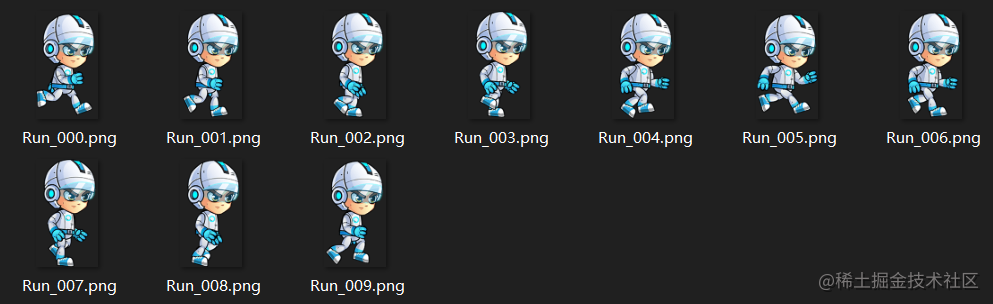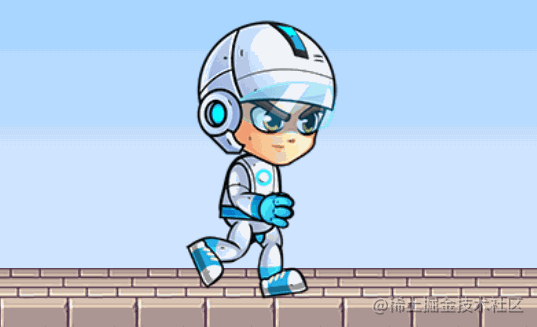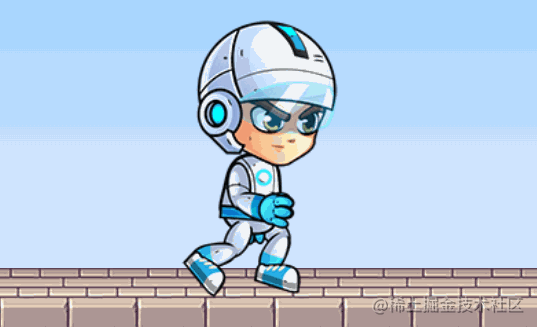``````for (let index = 0; index < 10; index++) {
let img = require('@/assets/images/user/run/Run_00'+index+'.png')
this.userRunList.push(img)
}
``````

``````// 动画
run() {
let _this = this
let _index = 0

let _run = () => {
let now = new Date().getTime()
if (now - this.lastRunTime > 120 - (this.speed * 20)) {
if (_index > (this.userRunList.length - 1) ) {
_index = 0
}
_this.userPic = this.userRunList[_index]
_index++
this.lastRunTime = now
}
this.runInterval = requestAnimationFrame(_run)
}
_run()
},
``````

``````slide () {
this.userPic = this.userSlidePic
},
jump () {
this.userPic = this.userJumpPic
}
``````
##### 障碍物开发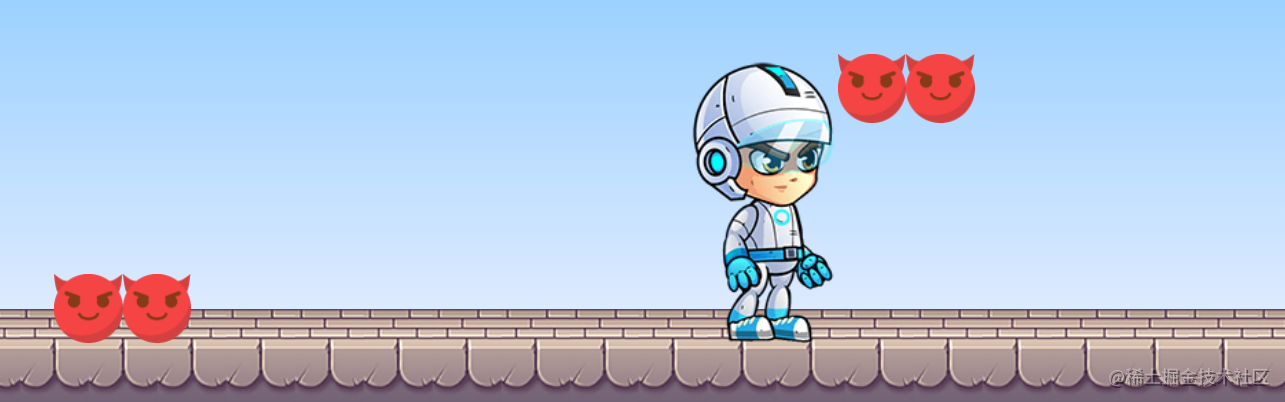``````// 生成障碍物
createObstruction () {
let obsList = document.createElement('div')
// 让障碍物随机在上方或者下方
let state = Math.random() > 0.5 ? 'top' : 'bottom'
obsList.className = 'obs-list-' + state
obsList.state = state
// 根据速度等级，随机生成相应数量的小恶魔
let random = Math.ceil(Math.random() * (this.speed - 2))
for (let index = 0; index < random; index++) {
let obsItem = document.createElement('div')
obsItem.className = 'obs-item'
obsList.appendChild(obsItem)
}
obsList.style.left = this.screenWidth + 'px'
// obsList.createNext = false // 是否已创建下一个障碍物
obsList.nextSpace = Math.random() * (this.obsInterval - this.obsInterval) + this.obsInterval // 下一个障碍物间隔

this.\$refs.obstructionWrap.appendChild(obsList)

},
``````

``````  // 获取所有障碍物
let obsDoms = this.\$refs.obstructionWrap.children
let obsList = Array.from(obsDoms)

let nextItem = null

// 给每个障碍物添加移动
for (let index = 0; index < obsList.length; index++) {
let item = obsList[index]
if (item.offsetLeft < -item.offsetWidth) {
this.\$refs.obstructionWrap.removeChild(item)
} else {
item.style.left = item.offsetLeft - this.speed * 3 + 'px'
}
}
``````

#### 玩法开发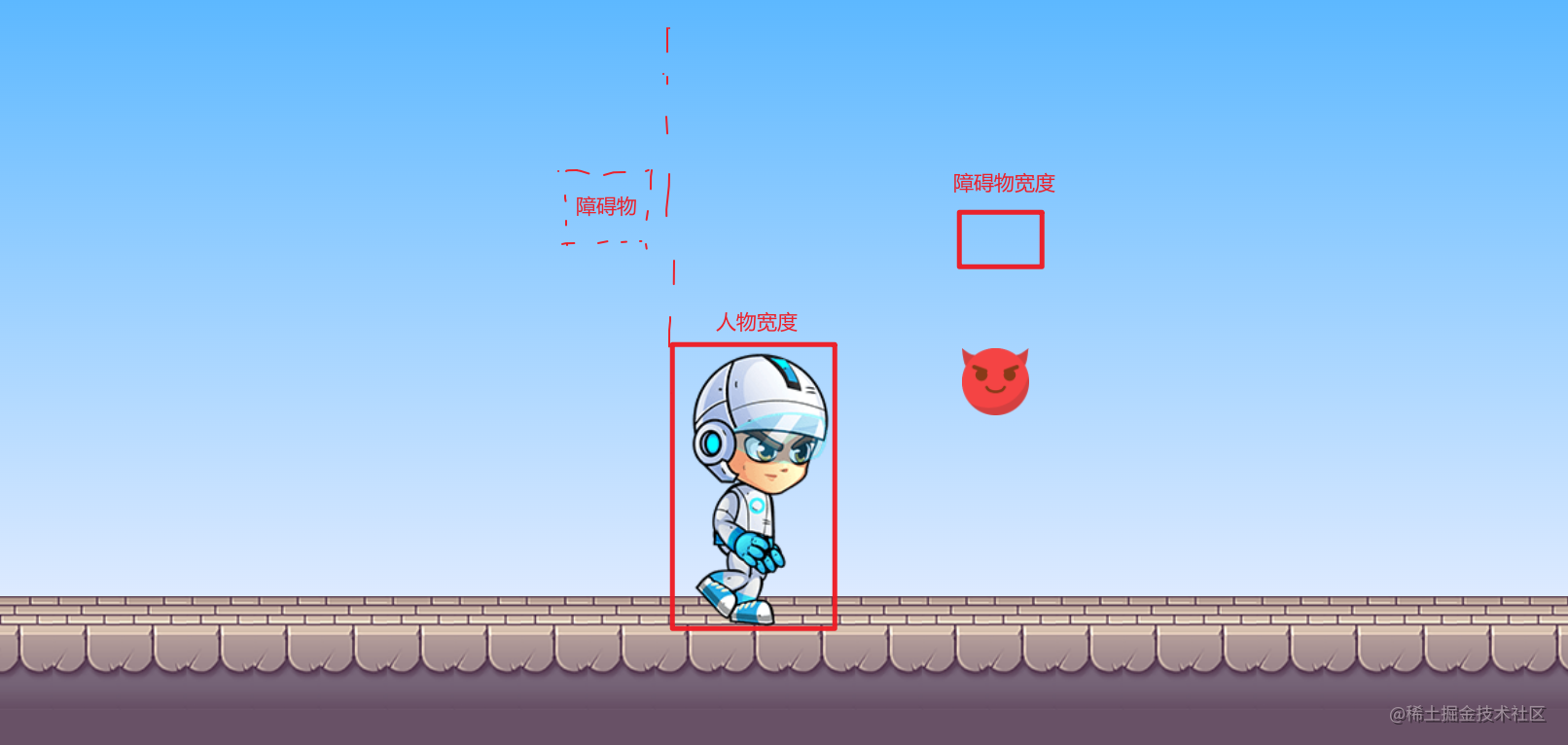``````let nextItem = null

// 给每个障碍物添加移动
for (let index = 0; index < obsList.length; index++) {
// 由于只需要找到第一个符合条件的障碍物即可，所以这里需要进行判断
if (!nextItem) {
// 找到人物右侧最近的障碍物，进行碰撞检测
// 需要进行检测的障碍物需满足条件：距离屏幕左侧距离>人物距离左侧距离+自身宽度
// 人物宽度为120，人物距离左侧距离为屏幕的一半减去自身的一半
if (item.offsetLeft > (this.screenWidth / 2 - 60 - item.offsetWidth)) {
nextItem = item
}
}
}
``````

``````// 碰撞检测
// 当距离最近的障碍物处于检测区时，进行碰撞检测
if (nextItem.offsetLeft < (this.screenWidth / 2 + 60)) {
if (nextItem.state === 'top') {
if (this.userStatus !== 'slide') {
this.\$emit('gameOver')
// 游戏结束
// this.gameOver()
return
}
} else {
if (this.userStatus !== 'jump') {
// 游戏结束
this.\$emit('gameOver')
//  this.gameOver()
return
}
}
}
``````

``````// 整体障碍物移动
obsMove () {
// 获取所有障碍物
let obsDoms = this.\$refs.obstructionWrap.children
let obsList = Array.from(obsDoms)

let nextItem = null

// 给每个障碍物添加移动
for (let index = 0; index < obsList.length; index++) {
let item = obsList[index]
if (item.offsetLeft < -item.offsetWidth) {
this.\$refs.obstructionWrap.removeChild(item)
} else {
item.style.left = item.offsetLeft - this.speed * 3 + 'px'
}

// 由于只需要找到第一个符合条件的障碍物即可，所以这里需要进行判断
if (!nextItem) {
// 找到人物右侧最近的障碍物，进行碰撞检测
// 需要进行检测的障碍物需满足条件：距离屏幕左侧距离>人物距离左侧距离+自身宽度
// 人物宽度为120，人物距离左侧距离为屏幕的一半减去自身的一半
if (item.offsetLeft > (this.screenWidth / 2 - 60 - item.offsetWidth)) {
nextItem = item
}
}
}

// 碰撞检测
// 当距离最近的障碍物处于检测区时，进行碰撞检测
if (nextItem.offsetLeft < (this.screenWidth / 2 + 60)) {
if (nextItem.state === 'top') {
if (this.userStatus !== 'slide') {
this.\$emit('gameOver')
// 游戏结束
// this.gameOver()
return
}
} else {
if (this.userStatus !== 'jump') {
// 游戏结束
this.\$emit('gameOver')
//  this.gameOver()
return
}
}
}

// 找到最后一个障碍物，创建下一个障碍物
let lastChild = obsList[obsList.length - 1]
// console.log(lastChild.nextSpace);
if (lastChild.offsetLeft < (this.screenWidth - lastChild.offsetWidth - lastChild.nextSpace)) {
this.createObstruction()
}
this.obsMoveInterval = requestAnimationFrame(this.obsMove)
},
``````